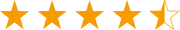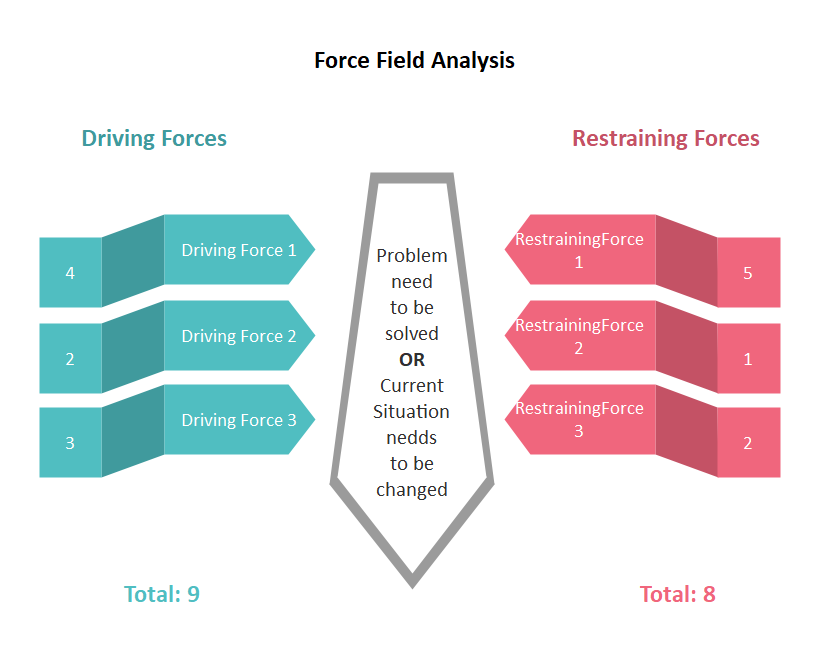A versatile cross-platform mind mapping tool.Get from App StoreA versatile cross-platform mind mapping tool.Get from App Store
edraw-max-horizontal
Product Tour
Guide
What's New
EdrawMax Online
TRY IT FREE

# Force Field Analysis Diagram

> Basic Diagram Knowledge > Force Field Analysis Diagram
Posted by James Freeman | 12/23/2019
Force Field Analysis Diagram is a simple but powerful tool for listing 2 kinds of forces that will drive and resist a proposed change.

The Force Field Analysis diagram is based on the work of social psychologist Kurt Lewin. According to him, human behavior is caused by forces – beliefs, expectations, cultural norms, and the like – within the "life space" of an individual or society. This may sound a little complicated to understand, but once put this theory into diagram, it would be much easier to follow.

### Explain Force Field Analysis Diagram with Example

Here is a Force Field Analysis diagram example for losing weight. Take a close look and we will elaborate on this.When creating a Force Field Analysis diagram, follow the steps:

1. Put one header (Force Field Analysis) at the center and two columns (two sets of forces) on each side,
2. Write the planned change in the central area,
3. Label the left column "driving forces", and the right one "restraining forces",
4. List the forces in the two columns,
5. Calculate the score each force has,

As shown in this example, a force field analysis diagram displays the driving forces and restraining forces that affect a question or problem needs to be solved. A force field analysis diagram can be used to compare forces in any situations from different points of view.

In this diagram of losing weight, driving forces could be described as the factors that push for change while restraining forces prevent this change. This is the goal of a force field analysis diagram, this diagram is used to analyze these forces to see whether a person should start changing. When the driving forces and restraining forces are equal, or the restraining forces are stronger than the driving forces, there would be no change. The change will only happen when the driving forces overcome the restraining forces.

### Other Uses of Force Field Analysis Diagram

The Force Field Analysis diagram is applicable to situations other than comparing driving and restraining forces. You can use a Force Field Analysis diagram to:

1. List pros and cons of a certain situation,
2. List actions and reactions for comparison,
3. List strength and weaknesses for personality analysis,
4. Compare the interests of two opposing parties in a negotiation,

#### You may also want to know

Examples for Force Field Diagram

Templates for Force Field Diagram

Force Field Analysis Diagram for PDF

Force Field Analysis Diagram for PPT

Force Field Analysis Diagram for MS Word

## Get Started! You Will Love This Easy-To-Use Diagram Software

EdrawMax is an advanced all-in-one diagramming tool for creating professional flowcharts, org charts, mind maps, network diagrams, UML diagrams, floor plans, electrical diagrams, science illustrations, and more. Just try it, you will love it!